Next: Resonance Up: One-Dimensional Motion Previous: Damped Oscillatory Motion

Quality Factor

The total energy of a damped oscillator is the sum of its kinetic and potential energies: i.e.,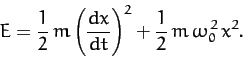(94)

Differentiating the above expression with respect to time, we obtain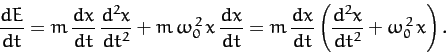(95)

It follows from Equation (83) that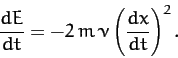(96)

We conclude that the presence of damping causes the oscillator energy to decrease monotonically in time, and, hence, causes the amplitude of the oscillation to eventually become negligibly small [see Equation (81)].

The energy loss rate of a weakly damped (i.e.,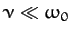) oscillator is conveniently characterized in terms of a parameter,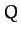, which is known as the quality factor. This parameter is defined to be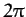times the energy stored in the oscillator, divided by the energy lost in a single oscillation period. If the oscillator is weakly damped then the energy lost per period is relatively small, andis therefore much larger than unity. Roughly speaking,is the number of oscillations that the oscillator typically completes, after being set in motion, before its amplitude decays to a negligible value. Let us find an expression for.

Now, the most general solution for a weakly damped oscillator can be written in the form [cf., Equation (91)]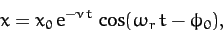(97)

where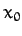and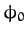are constants, and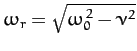. It follows that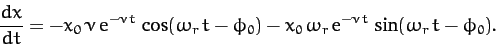(98)

Thus, making use of Equation (96), the energy lost during a single oscillation period is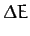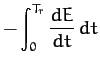(99)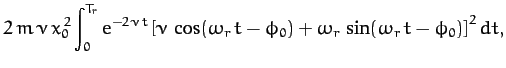where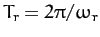. In the weakly damped limit,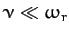, the exponential factor is approximately unity in the interval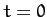to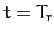, so that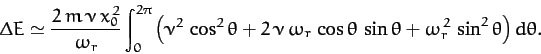(100)

Thus,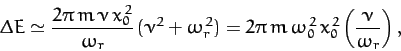(101)

since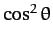and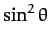both have the average values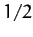in the interval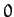to, whereas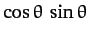has the average value. According to Equation (81), the energy stored in the oscillator (at) is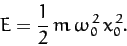(102)

It follows that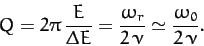(103)Next: Resonance Up: One-Dimensional Motion Previous: Damped Oscillatory Motion
Richard Fitzpatrick 2011-03-31